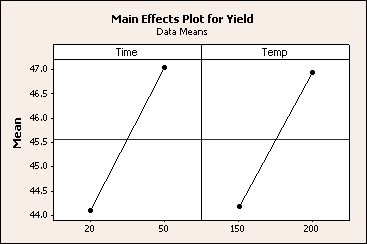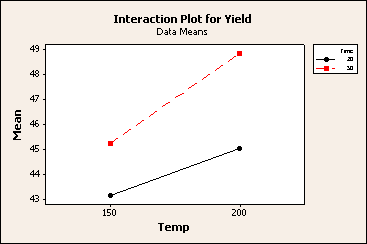# Least squares versus maximum likelihood estimation methods

tải về 0.49 Mb.
 trang 9/11 Chuyển đổi dữ liệu 06.06.2018 Kích 0.49 Mb.

## Least squares versus maximum likelihood estimation methods

Two different approaches for estimating population parameters from a random sample. The need to choose between these two methods primarily occurs in Minitab's reliability commands and Analyze Variability.

### Least squares method

Least squares estimates are calculated by fitting a regression line to the points from a data set that has the minimal sum of the deviations squared (least square error). In reliability analysis, this is plotted on a probability plot which can make interpretation easier.

### Maximum likelihood method

The likelihood function indicates how likely the observed sample is a function of possible parameter values. Therefore, maximizing the likelihood function determines the parameters that are most likely to produce the observed data. From a statistical point of view, MLE is generally recommended for large samples because it is versatile, applicable to most models and different types of data, and produces the most precise estimates.

### Comparison

In many cases, the differences between the LS and MLE results are minor, and the methods can be used interchangeably. You may want to run both methods and see whether the results confirm one another. If the results differ, you may want to determine why. Otherwise, you may want to use the more conservative estimates or consider the advantages of both approaches and make a choice for your problem. There are some areas where one approach has an advantage over the other:
 LSE MLE Biased No Yes for small samples but decreases as sample size increases Estimate Variance Larger Smaller P-values More precise Less precise Coefficients Less precise More precise Censored data Less reliable and unusable in extreme cases More reliable even in extreme cases

Use LSE when the sample size is small and censoring is not particularly heavy. Otherwise, MLE estimates are generally preferred. Based on their relative strengths, LSE and MLE can be used together for different parts of the analysis. Use LSE's more precise p-values to select the terms to include in the model and use MLE to estimate the final coefficients.

## Example of factorial plots

Trong thí dụ trên sự tương tác giữa thời gian và nhiệt độ là có ý nghĩa và chúng ta muốn nhìn biểu đố nhân tố (factorial plot) để giúp hiểu được bản chất của mối quan hệ . Vì ảnh hưởng của khối và chất xúc tác là không có ý nghĩa nên không đưa chúng vào biểu đồ.

1    Open the worksheet YIELDPLT.MTW. (The design, response data, and model information have been saved for you.)

2    Choose Stat > DOE > Factorial > Factorial Plots.

3    Check Main effects plot and click Setup.

4    In Responses, enter Yield.

5    Click to move Time to the Selected box.

6    Click to move Temp to the Selected box. Click OK.

7    Repeat steps 3-6 to set up the interaction plot. Click OK.

Graph window outputCơ sở dữ liệu được bảo vệ bởi bản quyền ©hocday.com 2019
được sử dụng cho việc quản lý

Quê hương